# Difference between revisions of "Test volume method"

An alternative to the virial pressure route to calculating the pressure, there is a method which consists on evaluating the change in internal energy,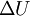$\Delta U$ produced by a small change in the volume of the system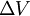$\Delta V$.
This should be tough of as a "blow-up" (or "in") of the whole system,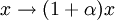$x \rightarrow (1+\alpha) x$ with a small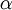$\alpha$ (same for y and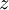$z$), exactly in the way as the virial pressure expression may be derived - not as leaving the particles in their places and changing the simulation cell (a procedure which does not make sense in general).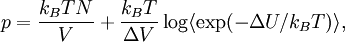$p = \frac{ k_B T N}{V} + \frac{ k_B T }{ \Delta V } \log \langle \exp(-\Delta U/k_B T )\rangle,$
where$k_B$ is the Boltzmann constant and$T$ is the temperature. The method is clearly inspired by the Widom test-particle method to obtain the chemical potential.